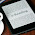### Steady State Analysis of AC and DC circuits - Topicwise GATE Questions on Network Theory (from 2003)

2005
1. For the circuit in figure, the instantaneous current i1(t) is

2007
2. In the AC network shown in the figure, the phasor voltage VAB(in volts) is:

2008
Common Data Questions: 3 & 4
The following series RLC circuit with zero initial conditions is excited by a unit impulse function δ(t).

3. For t > 0, the output voltage Vc(t) is

4. For t > 0, the voltage across the resistor is

Solution (3 & 4) : https://www.youtube.com/watch?v=nn6PggFjKgA

2009
5. The time domain behaviour of an RL circuit is represented as shown below. For an initial current of i(0) = Vo/R, the steady state value of the current is given by

2010
6. The current I in the circuit shown is

a) - j1 A
b) j1 A
c) 0 A
d) 20 A

2011
7. In the circuit shown below, the Norton equivalent current in amperes with respect to the terminals P and Q is

a) 6.4 - j 4.8
b) 6.56 - j 7.87
c) 10 + j 0
d) 16 + j 0

8. The circuit shown below is driven by a sinusoidal input Vi = Vpcos(t/RC). The steady state output Vo is

9. In the circuit shown below, the current I is equal to

2012
10. In the circuit shown below, the current through the inductor is

2014
11. In the circuit shown in the figure, the value of node voltage V2 (in volts) is

a) 22 + j 2
b) 2 + j 22
c) 22 – j 2
d) 2 – j 22

12. The steady state output of the circuit shown in the figure is given by y(t) = A(ω) sin(ωt + φ(ω)).

If the amplitude |A(ω)| = 0.25, then the frequency ω is

13. In the circuit shown in the figure,

the value of Vo(t) (in volts) for t → ∞ is ___________

2015
14. In the circuit shown, assume that diodes D1 and D2 are ideal. In the steady state condition, the average voltage Vab (in volts) across 0.5 µF capacitor is ____________

1.check out here for made easy aptitude book pdf if any one BARC question paper if possible to send me aakashvrm879@gmail.com

2.Name

Email *

Message *